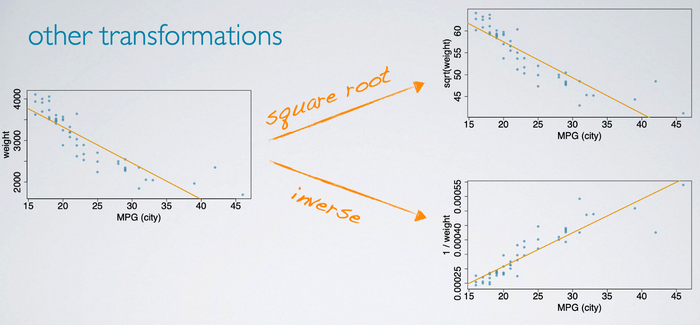### What is Data Transformations

Data Transformations are useful tricks for making certain types of data easier to model.

A transformation is a rescaling of the data using a function. When data are very stongly skewed, sometimes we transform them so they are easier to model.

### (Natural) log transformation

The most commonly used transformation is the natural log transformation. Natural log transformation is often applied when:

• Much of the data cluster near zero (relative to larger values in the data set).
• and, all observations are positive.
For example:

Here is the distributions of income per person, it is heavily right skewed, but after applying natural log transformation the data become much more symmetric. Sometimes this type of data are much easier to model because they are much less skewed and outliers are usually less extreme.Transformations can also be applied to one or both variables in scatterplot to make the relationship between variables more linear, and hence easier to model with simple methods.

For example:

Here is a scatterplot of income per person versus life expectation. The relationship is positive and curved, after applying a log transformation, the relationship stays positive but becomes more linear, which makes it easier to model than the untransformed data.#### Other transformations

In addition to the log, we can also try a square root transformation, where we plot the square root of the weight versus miles per gallon. Or the inverse transformation, where we divide 1 by the weight of the car.It is difficult to tell just looking at these plots which transformation works better, or if either of the transformations actually yield something better than the original data.

### Goals of Transformations

It is important to understand why we need to apply a transformation in the first place. So, here is the common goals in transforming data.

• To see the data structure differently
• To reduce skew assist in modeling
• To straighten a nonlinear relationship in a scatterplot so that we can model the relationship with simpler methods.